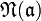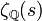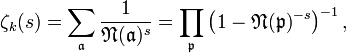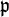# Dedekind zeta function

In mathematics, to each algebraic number field k, there is associated an important function called the Dedekind zeta function. This function is a generalization of the Riemann zeta function, and its definition is similar. Its importance stems from the fact that it encodes all of the important arithmetic information about the field in an analytic manner. As such, important results about the arithmetic in the field can be obtained from the associated zeta function through analytic methods.

## Definition

If k is an algebraic number field, the Dedekind zeta function of the field is a meromorphic function, defined for complex numbers s with real part satisfying$\Re (s) > 1$ by the Dirichlet series$\zeta_{k} (s) = \sum_{\mathfrak{a}} \frac{1}{\mathfrak{N} (\mathfrak{a})^{s}},$

where the sum extends over the set of integral ideals of k, and$\mathfrak{N} (\mathfrak{a})$ denotes their absolute norm.

This series is absolutely convergent on compact subsets of the half-plane$\Re (s) > 1$. It thus defines a holomorphic function on this half-plane, and this can be extended by analytic continuation to a meromorphic function on the whole complex plane. It is holomorphic everywhere except at s = 1, where it has a simple pole. The zeta function$\zeta_{\mathbb{Q}} (s)$ associated to the field of rational numbers is just the Riemann zeta function.

## Properties

The Dedekind zeta function has an Euler product:$\zeta_{k} (s) = \sum_{\mathfrak{a}} \frac{1}{\mathfrak{N} (\mathfrak{a})^{s}} = \prod_{\mathfrak{p}} \left(1-\mathfrak{N} (\mathfrak{p})^{-s}\right)^{-1} ,$

where$\mathfrak{p}$ runs over prime ideals of the ring of integers, which formally expresses the unique factorisation of ideals into prime ideals.

Just like the Riemann zeta function, each Dedekind zeta function possesses a functional equation. Furthermore, the analytic class number formula, which appears trivial in the context of the Riemann zeta function, expresses the residue of the pole at s = 1 in terms of the important arithmetic invariants of the field.Some content on this page may previously have appeared on Citizendium.